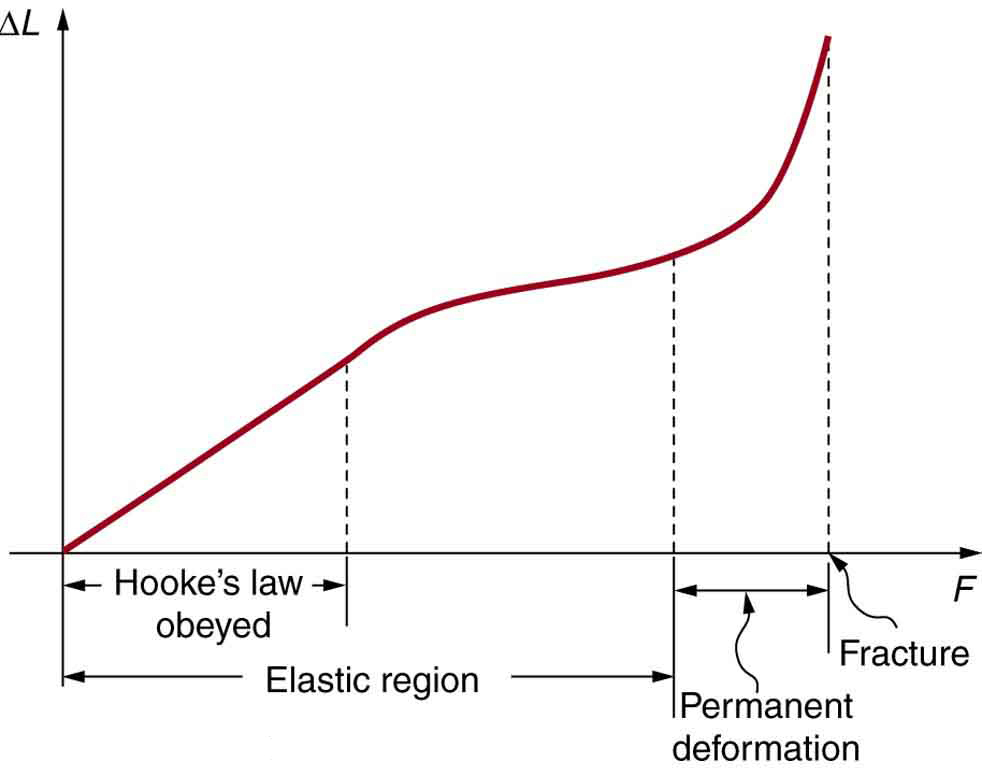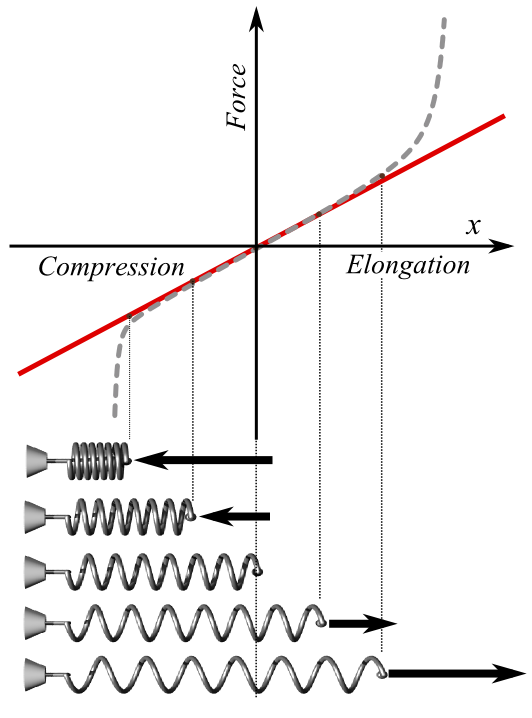# Materials Energy

• Astrophysics• Electricity• Electromagnetism• Energy• Fields• Force• Mechanics• Momentum• Nuclear Physics• Quantities & Units• States of Matter• Waves• Key ExperimentsMaterials are affected by forces like tension and compression, which require energy to work. This energy is mechanical and helps compress or expand the material, but only if it's elastic. When the material deforms, the work done to it is stored as energy, without changing its shape. This energy can be used later. So, materials and energy are closely related when it comes to deformation.

## What is the energy in a material?

When a material is compressed or elongated, the internal structure of the material compresses or elongates, too. Depending on the elasticity of the material, this results in more or less deformation. If the material is still in the elastic zone, the structure of the material will revert to its original state.

Figure 1 shows that when a force 'F' is applied to an elastic material, it elongates by a certain amount, represented by the symbol δl=a in the first image. If the material is still within its elastic zone after the force is removed (as indicated by the black cross in the second image), it means that the material has returned to its original length lo.

When the material is within its elastic zone, the energy used to stretch it by a certain length is called elastic strain energy. This energy can be recovered when the force is removed. However, if the material is deformed in the plastic area, this energy cannot be recovered. To calculate the energy used to deform the material, you only need to calculate the area under the curve.

### How to calculate the elastic strain energy

Because the elastic strain energy occurs in the elastic zone, the area below the curve for the elastic area can be calculated using the following formula:

Here, F is the maximum force where the material reaches its elastic limit, which is measured in Newtons, while Δl is the extension reached in metres.In the zone where the material has an elastic response (elastic zone), the elastic strain energy can be calculated if we know the force F and the elongation Δ

The main difference between this curve and the stress-strain curve is that the stress is the force per area while the strain is the deformation relative to the object’s original length. A metal bar is deformed by an increasing force. The material reaches its elastic limit where the relationship between the force and the elongation is not linear after deforming the bar by 10mm and the force reaches 7.5N. Calculate the elastic strain energy. As the material is being tested under elastic conditions, the relationship between the force and the deformation is a straight line. You can draw this line from the initial point where the force and the deformation are 0 to the point where F = 7.5N and s = 10 mm.In this example, the force reaches 7.5N, after which the material begins its plastic phase. The material reaches an elongation of 10mm

The area below the curve will be equal to half the area of the rectangle, with a height of F = 7.5 and a side of s = 10.

In the area where the relationship between the stress and the strain and the force and the extension is linear, the material follows Hooke’s law.

### Energy stored by a spring

Springs are commonly used in many different applications, from simple toys to machines and vehicles. When a spring is compressed or stretched, it stores potential energy that can be converted into kinetic energy when it is released. This is why springs are often used in devices such as shock absorbers, where the stored energy can help absorb the impact of a sudden force or movement. The ability of springs to store and release energy makes them a valuable component in many mechanical systems.

Sure! Let's begin by calculating the potential energy stored in the spring:

Potential Energy = ½kΔ²

where k is the spring constant and Δ is the elongation measured in metres.

In this case, k = 17 N/m and Δ = 0.05 m (5 cm converted to metres).

Potential Energy = 0.5 x 17 N/m x (0.05 m)²
Potential Energy = 0.010625 J

Now, let's calculate the velocity of the spring after it is released:

Kinetic Energy = ½mv²

where m is the mass of the spring and v is its velocity.

The potential energy stored in the spring is converted into kinetic energy when it is released, so:

Potential Energy = Kinetic Energy

0.010625 J = ½(0.1 kg)v²

v² = (2 x 0.010625 J) / 0.1 kg
v² = 0.2125 m²/s²
v = √(0.2125 m²/s²)
v = 0.461 m/s

Therefore, the velocity of the spring after it is released is approximately 0.461 m/s.We can also calculate the energy in the spring using the elastic strain energy as long the spring remains on its elastic limit

Energy related to elasticity in materials is a complex concept that involves the behavior of the material's structure under mechanical stress conditions. When a force stretches or compresses a material, it stores mechanical energy that can be released later. The elastic strain energy is the energy stored in a material when it is deformed elastically. This energy can be calculated by finding the area below the force vs elongation curve. This area is equal to the work done on the material to deform it elastically. The elastic strain energy can only be calculated in the elastic zone, which is the range of deformation where the material compresses or extends linearly proportional to the force acting on it, following Hooke's law. If the material is deformed beyond the elastic zone, the deformation becomes irreversible and the material may be permanently deformed.

## Materials Energy

What is the elastic strain energy in materials?

The elastic strain energy represents the work done on the material to compress or elongate it elastically. It is the area below the curve defined by the force and the elongation of the material as long this is within the elastic zone.

How do we calculate the energy stored in an elastic material?

To calculate the energy stored in an elastic material, we need to calculate the area below the linear relationship of the force and the elongation. To do this, we multiply the force by how much the material was deformed and divide it by 2. However, this is only valid for the elastic zone.

How are elastic materials and Hooke’s law related?

As Hooke’s law applies to materials with elastic properties, any elastic material that elongates or is compressed proportionally to the force applied follows Hooke’s law. This means that as long the force vs the elongation of any material follows a straight line with the slope ‘m’, we can say that those materials follow Hooke’s law.14-day free trial. Cancel anytime.Join 10,000+ learners worldwide.The first 14 days are on us96% of learners report x2 faster learningFree hands-on onboarding & supportCancel Anytime